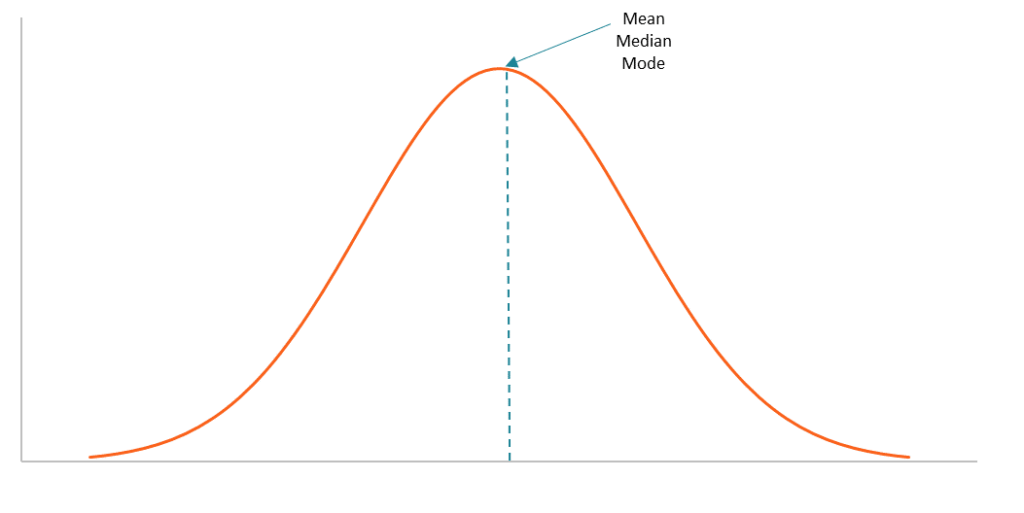# Bell Curve

A graph that depicts a normal probability distribution

# What is a Bell Curve?

A bell curve is an informal name of a graph that depicts a normal probability distribution. The term obtained its name due to the bell-shaped curve of the normal probability distribution graph.However, the term is not quite correct because the normal probability distribution is not the only probability distribution whose graph shows a bell-shaped curve. For instance, the graphs of the Cauchy and logistic distributions also demonstrate a bell-shaped curve.

### Characteristics of a Bell Curve

The bell curve is perfectly symmetrical. It is concentrated around the peak and decreases on either side. In a bell curve, the peak represents the most probable event in the dataset while the other events are equally distributed around the peak. The peak of the curve corresponds to the mean of the dataset (note that the mean in the normal probability distribution also equals the median and the mode).

The dispersion of the data on the bell curve is measured by the standard deviation. The probabilities of the bell curve and the standard deviation share a few important relationships, including:

• Around 68% of the data lies within 1 standard deviation.
• Around 95% of the data lies within 2 standard deviations.
• Around 99.7% of the data lies within 3 standard deviations.

The relationships described above are known as the 68-95-99.7 rule or the empirical rule. The empirical rule is primarily used to calculate the confidence interval of the normal probability distribution.

The concept is extremely important in statistics due to the wide applications of the normal probability distribution. For instance, the normal probability distribution is used as a representation of the distribution of random variables whose real distribution is unknown.

### Bell Curve in Finance

In finance, the normal probability distribution and the bell curve also play significant roles. Financial analysts frequently rely on the normal probability distribution in analyzing the returns of securities. The assumption of the normal distribution is fundamental in many pricing models that intend to predict future returns.

However, one must be careful with the normal probability distribution assumption in finance. In reality, the returns on many securities tend to demonstrate a non-normal distribution. For instance, some distributions are skewed or with a kurtosis that is different from that of a normal distribution.